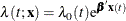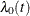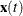### The PHREG Procedure

The PHREG procedure fits the proportional hazards model of Cox (1972, 1975) to survival data that might be right censored. The Cox model is a semiparametric model in which the hazard function of the survival time is given bywhereis an unspecified baseline hazard function,is a vector of covariate values (possibly time-dependent), andis a vector of unknown regression parameters. The model is referred to as a semiparametric model because part of the model involves the unspecified baseline function over time (which has an infinite dimension) and the other part involves a finite number of regression parameters. Several texts that discuss the Cox regression models are Collett (1994); Cox and Oakes (1984); Kalbfleisch and Prentice (1980); Lawless (1982). Extensions of the Cox model are discussed in Therneau and Grambsch (2000); Andersen et al. (1992); Fleming and Harrington (1991). For more information about PROC PHREG, see Chapter 67: The PHREG Procedure.